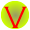## Monday 14 November 2011

### NTSE SAT Quiz-7 (Maths)

Q1: The sides of a right triangle are a, a+d, a+2d with a and d both positive. What is the value of ratio a:d ?
(a) 1: 3
(b) 1:4
(c) 2:1
(d) 3:1

Q2: In a group of cows and hens, the number of legs was 14 more than twice the number of heads. the number of cows is:
(a) 5
(b) 7
(c) 12
(d) 14

Q3: Each edge of cube is increased by 50%. The percent increase in surface area of the cube will be:
(a) 50
(b) 125
(c) 150
(d) 300

Q4: Evaluate $(0.000343)^\frac{1}{3}$
(a) 7/100
(b) 100/7
(c) 7/10
(d) 10/7

Q5: A student was asked to multiply a given number by 4/5. By mistake he divided it by 4/5. His answer was 36 more than the correct answer.  Find the given number.
(a) 80
(b) 60
(c) 36
(d) 63

Q6: A number is divisible by 3, if
(a) the last digit is 3
(b) the last digit is 6
(c) the last two digits are 3
(d) sum of digits is divisible by 3

Q7: A number lies between 1000 and 2000. On division by 2,3,4,5,6, 7 and 8 it leaves remainders 1,2,3,4,5,6 and 7 respectively. The number is:
(a) 1876
(b) 1778
(c) 1679
(d) 1654

Q8: 1400 men in a fortress have provisions for 28 days. After 3 days, 400 men leave the fortress. How long will the food last now?
(a) 35 days
(b) 32 days
(c) 30 days
(d) 28 days

Q9: In a parallelogram ABCD the ratio between angles $\angle A$ and $\angle B$ is 1:2. Find the $\angle A$ ?
(a) 60
(b) 90
(c) 90
(d) 180

Q10: The ratio of the volumes of cylinder and cone of equal radii and height is:
(a) 1:3
(b) 3:1
(c) 1:1
(d) 1:2

1-d (Hint apply Pythagoras theorem and factorize the equation)
2-b
3-b (Hint: surface area of cube is $6d^2$
4-a
5-a
6-d
7-c (Hint: LCM of divisors is 840, hence 840x-1 represent the number. Find x. )
8-a
9-a
10-b1.in q)7 how do we arrive at the expression 840x-1?

1.LCM of 2,3,4,5,6,7 and 8 is 840. If we subtract 1 from 840 i.e. 839 and divide it with divisors, we get remainder 1,2,3,4,5,6 and 7 respectively.
Since the number lies between 1000 and 2000. So number shall be multiple of 840 minus 1 i.e. 840x -1.
In this case 840*2 = 1680-1 = 1679
Hope it helps!

2.Finding Squares and Cube of First 20 Natural Numbers and Developing 2 SQUARE PATTERNS.